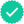#### Related questions#### Concept explainers

Question

Suppose, household color TVs are replaced at an average age of μ = 7.4 years after purchase, and the (95% of data) range was from 5.4 to 9.4 years. Thus, the range was 9.4 − 5.4 = 4.0 years. Let x be the age (in years) at which a color TV is replaced. Assume that x has a distribution that is approximately normal.

(a) The empirical rule indicates that for a symmetric and bell-shaped distribution, approximately 95% of the data lies within two standard deviations of the mean. Therefore, a 95% range of data values extending from μ − 2σ to μ + 2σ is often used for "commonly occurring" data values. Note that the interval from μ − 2σ to μ + 2σ is 4σ in length. This leads to a "rule of thumb" for estimating the standard deviation from a 95% range of data values.
Estimating the standard deviation

For a symmetric, bell-shaped distribution,
standard deviation ≈
 range 4
 high value − low value 4
where it is estimated that about 95% of the commonly occurring data values fall into this range.
Use this "rule of thumb" to approximate the standard deviation of x values, where x is the age (in years) at which a color TV is replaced. (Round your answer to one decimal place.)
yrs

(b) What is the probability that someone will keep a color TV more than 5 years before replacement? (Round your answer to four decimal places.)

(c) What is the probability that someone will keep a color TV fewer than 10 years before replacement? (Round your answer to four decimal places.)

(d) Assume that the average life of a color TV is 7.4 years with a standard deviation of 1.0 years before it breaks. Suppose that a company guarantees color TVs and will replace a TV that breaks while under guarantee with a new one. However, the company does not want to replace more than 14% of the TVs under guarantee. For how long should the guarantee be made (rounded to the nearest tenth of a year)?
yrs
Expert SolutionKnowledge BoosterRecommended textbooks for you
•A First Course in Probability (10th Edition)
Probability
ISBN:9780134753119
Author:Sheldon Ross
Publisher:PEARSONA First Course in Probability
Probability
ISBN:9780321794772
Author:Sheldon Ross
Publisher:PEARSON
•A First Course in Probability (10th Edition)
Probability
ISBN:9780134753119
Author:Sheldon Ross
Publisher:PEARSONA First Course in Probability
Probability
ISBN:9780321794772
Author:Sheldon Ross
Publisher:PEARSON